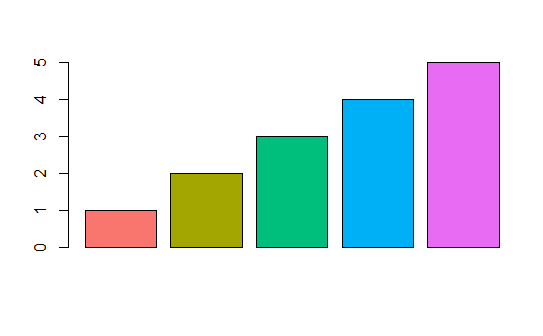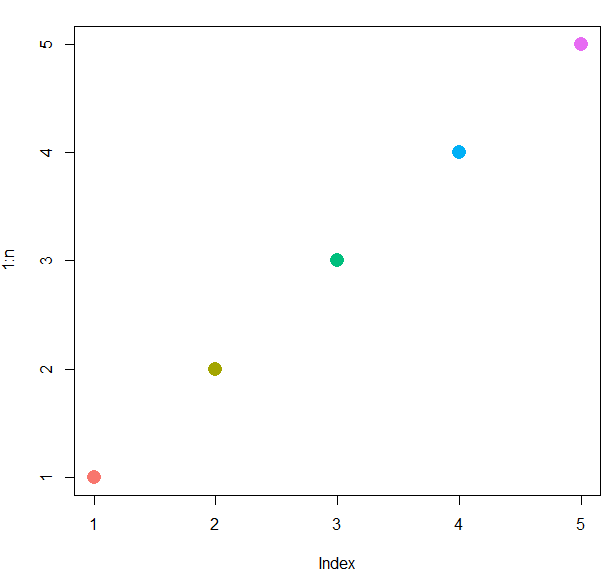2 views

What function can I use to emulate ggplot2's default color palette for a desired number of colors. For example, an input of 3 would produce a character vector of HEX colors with these colors:by

The default color scheme in ggplot2 i.e., scale_color_hue picks evenly spaced hues around the hcl (Hue-Chroma-Luminance) color wheel starting from 15.

You can emulate these colors using the following function:

gg_color <- function(n) {

hues = seq(15, 375, length = n + 1)

hcl(h = hues, l = 65, c = 100)[1:n]

}

For example:

To plot a bar plot by emulating 5 ggplot colors:

n = 5

cols = gg_color(n)

y <- 1:5

barplot(y, col = cols)

Output:OR
n = 5
cols = gg_color(n)
dev.new(width = 5, height = 5)
plot(1:n, pch = 16, cex = 2, col = cols)